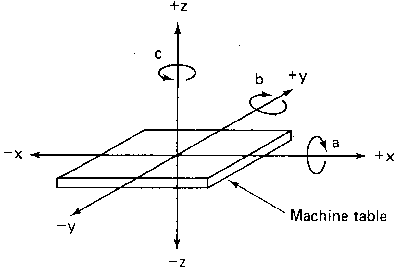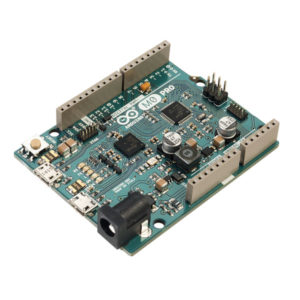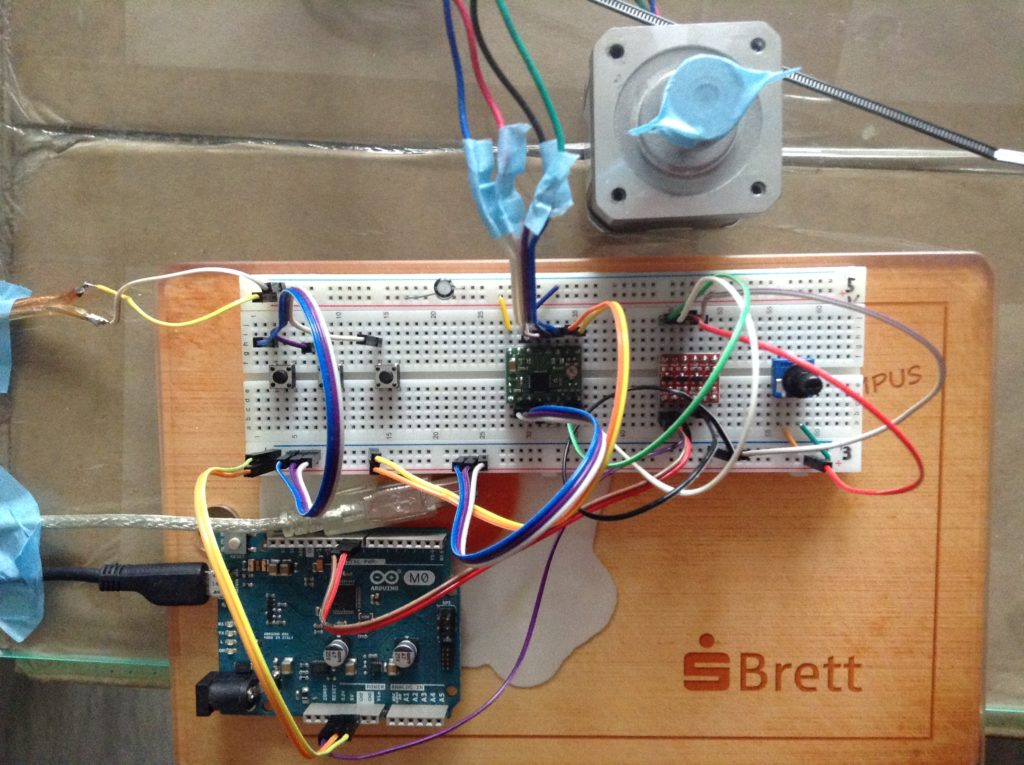# Virtual Work

So We’ve talked about mdc equations before in math monkey. They are usually in the form $$m\: \ddot{x} + d\: \dot{x} + cx\: = 0\:$$

m Being the Mass, d the dissapation (dampening) and c the spring constants.

I won’t go into how one builds this equation up, there are many and I covered in math monkey pretty in depth on how to do it with the basic math pendel. One of these days I may get into a more indepth analysis of maybe a car or a real double pendle. Anyways, a part that wasn’t explained at all was the “Virtual Work” of the system, or namely the $Q\:$ part of the Lagrange equation

$$\mathrm{Q}=\frac{d}{dt}\frac{\delta\mathrm{L}}{\delta\mathrm{\dot{\phi}}}-\frac{\delta\mathrm{L}}{\delta\mathrm{\phi}}$$

So whats Q? Well according to wiki:

Virtual work arises in the application of the principle of least action to the study of forces and movement of a mechanical system. The work of a force acting on a particle as it moves along a displacement will be different for different displacements.

Or whatever that means. In mathemagics, it means this:

$$Q\:= \displaystyle\sum_{i=1}^{n}\mathrm{M_i(t)}\frac{\delta\phi}{\delta\mathrm{x}}+\mathrm{F_i(t)}\frac{\delta\mathrm{r}}{\delta\mathrm{x}}+\mathrm{F_{di}(t)}\frac{\delta\mathrm{r_d}}{\delta\mathrm{x}}+\mathrm{R_i(t)}\frac{\delta\mathrm{r}}{\delta\mathrm{x}}$$

Namely the Sum of the Moment, external force, friction and dampening times their directional vector components first derivative. Or Well thats what it looks like when I read it anyways. I still haven’t quite got the hang/understanding of it. But I’ve never claimed to know what I’m doing. Just that I look like I know what I’m doing 😉

Well to calculate all this out theres quite a bit of work to do. I recently had to do such a thing in a system I had to describe and while digging through my notes I happened to fall on a trick I seemed to write to myself in a exam cheatsheet. Normally, you would calculate the vectoral force (for dampening in this example) and multiple it with its directional derivative like so:

$$\mathrm{F_{di}(t)}\frac{\delta\mathrm{r_d}}{\delta\mathrm{x}}$$

F could look like this (or rather, does):

$$\mathrm{\underline{F}_{d}(t)} = -d \dot{x} \vec{e_x}$$

and r would look like maybe this:

$$\delta\mathrm{r} = x\vec{e_x} +y \vec{e_y}$$

So you can imagine there are a bunch of steps to get this done, each partial div. blah blah multiplying, blah blah.

Well good news everyone!

I guess it’s not a surprise cause I already said I found a cheat. Well here it is:

$$\mathrm{\underline{F}_{d}(t)} = -d \Delta\Delta\dot{x} = -d \Delta\dot{x}^2$$

So if you’ve already kinematically described your system, You can take the derivatives you already know, square them, add them together and multiply by -d and you have your answer immediately.

Yay! I’ve tested this conjecture exactly once with 100% success rate. So that’s good math in my books. I wish I could credit where I found this from…but since it’s on my cheat sheet. I guess I’ll have to claim it as my own.

$\mathrm{e}^{\sqrt{2}}$

# Mighty Max Lives 2/3

I’ve been workin’ away on projects and being a half way good student…but terrible person. But in the meantime I’ve managed to get something done on Mighty Max. My Smoothieboard 0X showed up finally and I got straight to work on putting in in place.

# Mighty Max C-Axis and Igus

I mentioned in Accelstepper that I had been working on a 5 Axis platform for a laser cutting project. Well I’ve sent the drawings in and parts are slowly starting to come! We’ve already ordered and have many of the smaller parts…motors, drivers, (not yet controller….that’ll be another post) and the rotary parts for our rotational axis Namely the C-axis…Remember the ABC are the ones the rotate around our XYZ.

# Accelstepper

For work I’ve been designing a 5 axis laser cutter and since all machines deserve a name I deemed the machine to be called The Mighty Max Its from one of those shows I used to watch and buy toys from as a child where I’m not sure if the accompanying toys came before or after the show. Anyways. We’ve basically completely finished the C axis…which is the rotational axis around the Z axis in a Cartesian coordinate system like so:And we’d like to start testing initial designs to ensure we’ve actually got something going on here. So I decided to break out the arduino and some other little hardware bits to see if I can get something going and here’s my experience doing it.

So like a good little science person, one should have an idea to follow.

What do we want to do?

• Test stepper motors
• Count steps
• be able to send requests for steps
• Adjust speed and or acceleration
• must be usable with any kind of step/dir driver

So the easiest way to do any of this is really using an arduino and someone elses stepper library. Which thankfully one exists! The Accelstepper library which supports all sorts of banana things, but most interestingly is that it right off the bat crosses off three things off the wanted list.

• Test stepper motors
• Count steps
• be able to send requests for steps
• Adjust speed and or acceleration
• must be usable with any kind of step/dir driver

The other two things I want are just arduino things. I have an arduino M0 at home, which is a 32bit Uno shape/style boardSo those are gone to.

This is my code for the arduino m0…We include the Accelstepper library set it’s max speed, accel and set the serial speed aswell (since the m0 is so super fast, we can easily afford to bump the baudrate…

#include AccelStepper.h; // Define a stepper and the pins it will use AccelStepper stepper(1, 9, 8); long steps = 0; void setup() { stepper.setMaxSpeed(51200); //steps per second stepper.setAcceleration(8000); // stepper.setSpeed(9600); SerialUSB.begin(115200); } void loop() { if (SerialUSB.available() > 0) { steps = SerialUSB.parseInt(); SerialUSB.println(steps); stepper.moveTo(steps); while (stepper.distanceToGo() != 0) { // Serial.println(stepper.currentPosition()); stepper.run(); } } //Serial.write("hello"); }

This will let me with arduinos Serial monitor in the IDE send a command at how many steps I want to move in one direction or another, count them, move with accel in that direction and then let me go back to 0 and hopefully go back to its original position. (it does)

Here’s a video and some pictures on the breadboard# Exciting research day

Today I’ll be experimenting on a “ideal magnetic kreis” mainly to see if a person can get any kind of worthwhile pull in one direction or another from my super magnet.

Magnetics itself is a phenominoooo—…thing that has always interested me…actually probably like any science person. You can have a force interact with an object straight through the air without ever having to touch it. It’s pretty damn cool really.

Posted in R&D